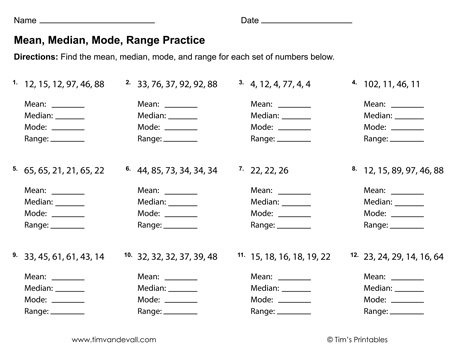# Mean, Median, Mode, Range Worksheets PDF | 3 Printable WorksheetsTeach your students about mean, median, mode and range with this printable practice math worksheets. There are 3 worksheets all together. In the first two worksheets, students are asked to find the mean, median, mode, and range for each set of numbers. In the third worksheet, students are asked to answer a set of questions based upon the chart.

Answer keys included on pages 4-6. PDF format.